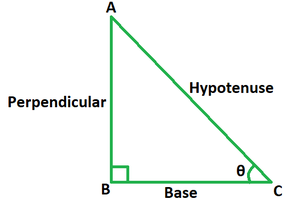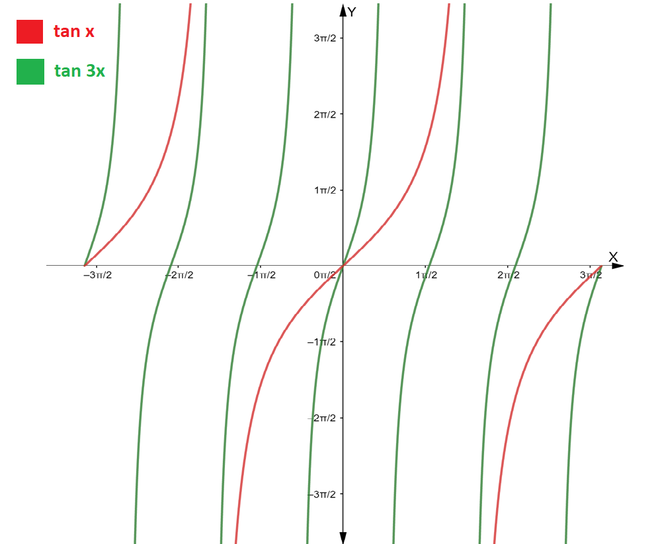# Tangent 3 Theta Formula

• Last Updated : 31 May, 2022

Trigonometry is the study of the connection between the ratios of the sides of a right-angled triangle and their angles. Various trigonometric ratios such as sine, cosine, tangent, cotangent, secant, and cosecant are used to examine this field of study. Trigonometry is formed from the names ‘Trigonon’ and ‘Metron,’ which represent a triangle and a measurement, respectively. It is the study of the connection between the sides and angles of a right-angled triangle. It, therefore, assists in calculating the unknown dimensions of a right-angled triangle by using equations and identities based on this relation.

### Tangent Trigonometric Ratio

The ratio of any two right triangle sides is called a trigonometric ratio. The tangent ratio is defined as the ratio of the length of the opposite side of an angle divided by the length of the adjacent side.If θ is the angle created by the base and hypotenuse of a right-angled triangle then,

tan θ = Perpendicular/Base = sin θ/ cos θ

Here, perpendicular is the side opposite to the angle and base is the side adjacent to it.

### Tangent 3 Theta (Tan 3θ) Formula

Tan3θ is a triple angle identity in trigonometry. It is a crucial trigonometric identity that is used to solve a variety of trigonometric and integration issues. It is a trigonometric function that returns the tan function value for a triple angle. It may alternatively be written as tan3θ = sin 3θ/cos 3θ since the tangent function is a ratio of the sine and cosine functions. The value of tan3θ repeats after every π/3 radians, tan3θ = tan (3θ + π/3). Its graph is thinner than tan θ’s graph.Derivation

The formula for Tangent 3 theta is derived by using the sum angle formula for Tangent theta and Tangent 2 theta ratios.

To demonstrate that tan 3θ = (3 tan θ – tan3θ) / (1 – 3 tan2θ), we write 3θ as (2θ + θ).

L.H.S. = tan 3θ

= tan (2θ + θ)

Use the formula tan (x + y) = (tan x + tan y) / (1 – tan x tan y)

= (tan 2θ + tan θ)/ (1 – tan 2θ tan θ)

Use the formula tan 2x = (2 tan x) / (1 – tan2x) for tan 2θ.

= [(2 tan θ / (1 – tan2θ)) + tan θ] / [1 – (2 tan θ / (1 – tan2θ)) tan θ]

= (tan θ – tan3θ + 2 tan θ) / (1 – tan2θ – 2 tan2θ)

= (3 tan θ – tan3θ) / (1 – 3 tan2θ)

= R.H.S.

This derives the formula for tangent 3 theta ratio.

### Sample Problems

Problem 1. If tan θ = 3/4, find the value of tan 3θ using the formula.

Solution:

We have, tan θ = 3/4.

Using the formula we get,

tan 3θ = (3 tan θ – tan3θ) / (1 – 3 tan2θ)

= (3 (3/4) – (3/4)3) / (1 – 3 (3/4)2)

= (9/4 – 27/64) / (1 – 3 (9/16))

= (117/64) / (-11/16)

= -117/44

Problem 2. If tan θ = 12/5, find the value of tan 3θ using the formula.

Solution:

We have, tan θ = 12/5.

Using the formula we get,

tan 3θ = (3 tan θ – tan3θ) / (1 – 3 tan2θ)

= (3 (12/5) – (12/5)3) / (1 – 3 (12/5)2)

= (36/5 – 1728/125) / (1 – 3 (144/25))

= (-828/125) / (-407/25)

= 828/2035

Problem 3. If sin θ = 4/5, find the value of tan 3θ using the formula.

Solution:

We have, sin θ = 4/5.

Clearly cos θ = 3/5. Hence we have, tan θ = 4/3.

Using the formula we get,

tan 3θ = (3 tan θ – tan3θ) / (1 – 3 tan2θ)

= (3 (4/3) – (4/3)3) / (1 – 3 (4/3)2)

= (4 – 64/27) / (1 – 3 (16/9))

= (44/27) / (-13/3)

= -44/117

Problem 4. If cos θ = 12/13, find the value of tan 3θ using the formula.

Solution:

We have, cos θ = 12/13.

Clearly sin θ = 5/13. Hence we have, tan θ = 5/12.

Using the formula we get,

tan 3θ = (3 tan θ – tan3θ) / (1 – 3 tan2θ)

= (3 (5/12) – (5/12)3) / (1 – 3 (5/12)2)

= (5/4 – 125/1728) / (1 – 3 (25/144))

= (2035/1728) / (19/144)

= 2035/228

Problem 5. If sec θ = 17/8, find the value of tan 3θ using the formula.

Solution:

We have, sec θ = 17/8.

Find the value of tan θ using the formula sec2 θ = 1 + tan2 θ.

tan θ = √((289/64) – 1)

= √(225/64)

= 15/8

Using the formula we get,

tan 3θ = (3 tan θ – tan3θ) / (1 – 3 tan2θ)

= (3 (15/8) – (15/8)3) / (1 – 3 (15/8)2)

= (45/8 – 3375/1728) / (1 – 3 (225/64))

= (72/25) / (64/675)

= 243/8

Problem 6. Find the value of tan 135° using the tan 3x formula.

Solution:

We have to find the value of tan 135°.

Let us take 3x = 135

=> x = 135/3

=> x = 45°

We know, tan 45° = 1.

Using the tan 3x formula, we get

tan 135° = (3 tan 45° – tan345°) / (1- 3 tan245°)

= (3(1) – 13) / (1 – 3 (12))

= (3 – 1) / (1 – 3)

= 2 / (-2)

= -1

Problem 7. Find the value of tan 75° using the tan 3x formula.

Solution:

We have to find the value of tan 75°.

Let us take 3x = 75

=> x = 75/3

=> x = 25°

We know, tan 25° = 0.47.

Using the tan 3x formula, we get

tan 75° = (3 tan 25° – tan325°) / (1- 3 tan225°)

= (3(0.47) – (0.47)3) / (1 – 3 (0.47)2)

= (1.41 – 0.10) / (1 – 3 (0.22))

= (1.31) / (0.34)

= 3.85

My Personal Notes arrow_drop_up